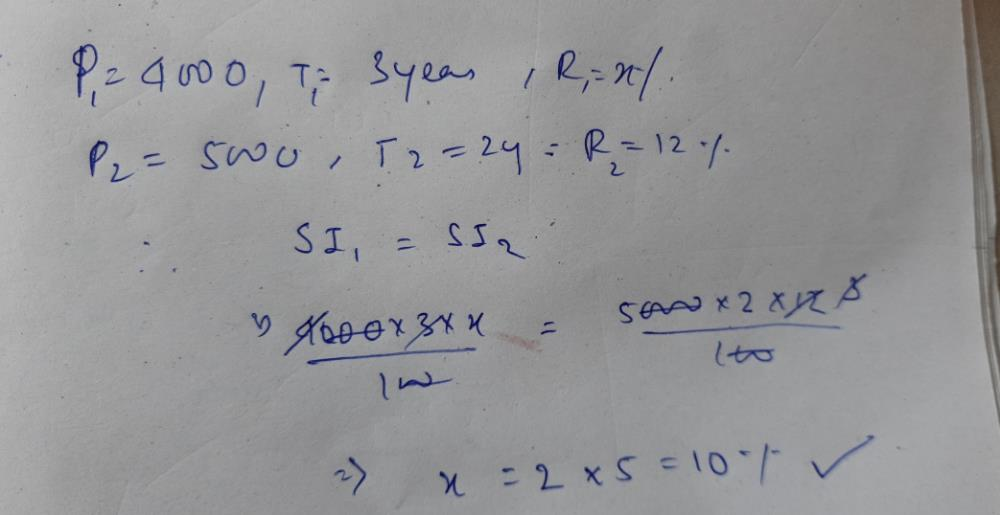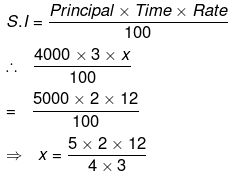Courses

# Solve the following questionThe simple interest on Rs. 4000 in 3 years at the rate of x% per annum equals the simple interest on Rs. 5000 at the rate of 12% per annum in 2 years. The value of x is (in %, in numerical value)Correct answer is '10'. Can you explain this answer? Related Test: CAT Mock Test - 9 (New Pattern)

## CAT QuestionKomal Verma 4 weeks ago
(4000*3*x)/100=(5000*12*2)/100
120x=1200
x=10Kirti Soni 4 weeks agoTalent Skill Learning 4 weeks ago= 10 % per annum